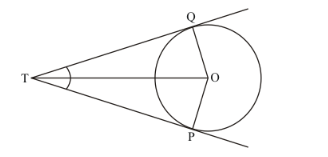# If TP and TQ are two tangents to a circle with centre O so that

Question:

If TP and TQ are two tangents to a circle with centre O so that ∠POQ = 110°, then, ∠PTQ is equal to

(a) 60°

(b) 70°

(c) 80°

(d) 90°

Solution:

Let us first put the given data in the form of a diagram.It is given that,

$\angle P O Q=110^{\circ}$

We have to find $\angle P T Q=110^{\circ}$

We know that the radius of the circle will always be perpendicular to the tangent at the point of contact. Therefore,

$\angle O Q T=90^{\circ}$

$\angle O P T=90^{\circ}$

Also, we know that sum of all angles of a quadrilateral will always be equal to $360^{\circ}$. Therefore in quadrilateral TQOP, we have,

$\angle O P T+\angle O Q T+\angle P T Q+\angle P O Q=360^{\circ}$

$90^{\circ}+90^{\circ}+110^{\circ}+\angle P O Q=360^{\circ}$

$290^{\circ}+\angle P O Q=360^{\circ}$

$\angle P O Q=70^{\circ}$

Therefore, the correct answer to this question is choice (b).